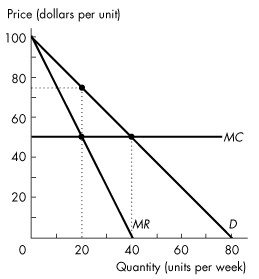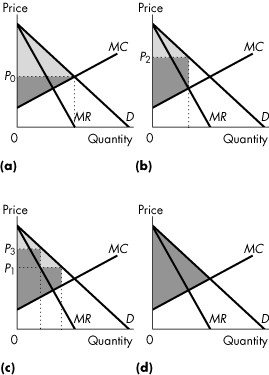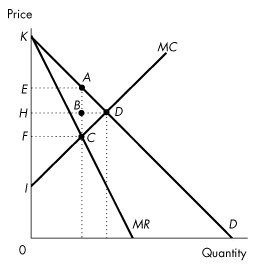/
/
/
11) Table 13.4.1 shows the demand schedule faced by a
Not my Question
Flag Content

# Question : 11) Table 13.4.1 shows the demand schedule faced by a : 1418011

11) Table 13.4.1 shows the demand schedule faced by a perfect price-discriminating monopoly. If 3 units are sold, total revenue is

A) \$15.00.

B) \$16.00.

C) \$18.00.

D) \$19.50.

E) \$5.00.

12) The output of a (not perfect) price-discriminating monopoly is

A) less than a single-price monopoly.

B) more than a single-price monopoly but less than a perfectly competitive industry.

C) the same amount as a perfectly competitive industry.

D) more than a perfectly competitive industry.

E) less than a single-price monopoly but more than a perfectly competitive industry.

13) A perfect price-discriminating monopoly produces

A) less than a single-price monopoly.

B) more than a single-price monopoly but less than a perfectly competitive industry.

C) less than a monopoly that practices price discrimination but not perfect price discrimination.

D) more than a perfectly competitive industry.

E) the same amount as a perfectly competitive industry.

14) A perfect price-discriminating monopoly

A) has a demand curve that is also its marginal revenue curve.

B) maximizes total revenue.

C) is inefficient.

D) will produce the quantity at which the marginal cost curve intersects its demand curve.

E) both A & D are correct.

15) Which of the following quotes by a store manager describes price discrimination in action?

A) "Since bulk goods are cheaper to package, I can offer a lower price."

B) "I can get a 4-litre jug of milk from the supplier for less than four 1-litre cartons, so I can sell it for less per litre."

C) "We offer our employees a 10 percent price discount."

D) "I offer discounts if you buy 12 apple juices in one box, although it costs me the same as if I split it up and sell them separately."

E) "We set price equal to average variable cost."

Use the figure below to answer the following question.Figure 13.4.3

16) Refer to Figure 13.4.3. The outcome is efficient if

A) the monopoly is able to perfectly price discriminate.

B) the price consumers pay is equal to average total cost.

C) the price consumers pay exceeds minimum average variable cost.

D) the quantity produced is 80 units.

E) the quantity produced is 20 units.

17) A perfect price-discriminating monopoly is

A) less efficient than a single-price monopoly.

B) more efficient than a single-price monopoly, but less efficient than a perfectly competitive industry.

C) as efficient as a single-price monopoly.

D) more efficient than a perfectly competitive industry.

E) as efficient as a perfectly competitive industry.

Use the figure below to answer the following questions.Figure 13.4.4

18) Refer to Figure 13.4.4. Consider a market with a monopoly that distinguishes between two groups of buyers. If the light grey area shows the consumer surplus and the dark grey area shows the producer surplus, which graphs correctly represents this firm?

A) (a)

B) (b)

C) (c)

D) (d)

E) (b) and (d)

19) Refer to Figure 13.4.4. Consider a market with a perfect price-discriminating monopolist. If the light grey area shows the consumer surplus and the dark grey area shows the producer surplus, which graph correctly represents this firm?

A) (a)

B) (b)

C) (c)

D) (d)

E) none of the graphs

Use the figure below to answer the following questions.Figure 13.4.5

20) Consider the cost and revenue curves in Figure 13.4.5. If this is a perfect price-discriminating monopoly, what is consumer surplus?

A) EADH

B) EABH

C) ABD

D) KEA

E) None of the above.

## Solution 5 (1 Ratings )

Solved
Economics 1 Year Ago 32 Views
This Question has Been Answered!